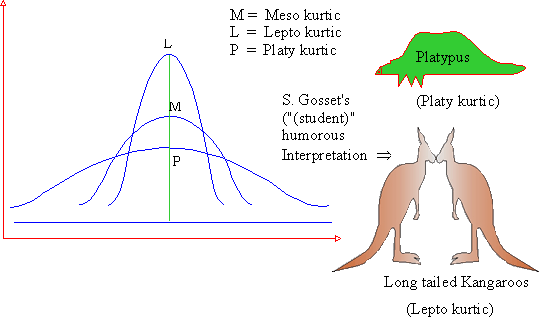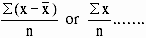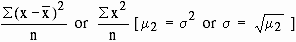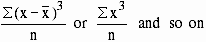Support the Monkey! Tell All your Friends and TeachersHome MonkeyNotes Printable Notes Digital Library Study Guides Study Smart Parents Tips College Planning Test Prep Fun Zone Help / FAQ How to Cite Request a New Title

5.10 Kurtosis

It has its origin in the Greek word "Bulginess." In statistics it is the degree of flatness or peakedness in the region of mode of a frequency curve. It is measured relative to the peakedness of the normal curve. It tells us the extent to which a distribution is more peaked or flat-topped than the normal curve. If the curve is more peaked than a normal curve it is called Lepto Kurtic. In this case items are more clustered about the mode. If the curve is more flat-toped than the more normal curve, it is Platy-Kurtic. The normal curve itself is known as "Meso Kurtic."Click here to enlarge

Moments

Moment is a familiar mechanical term for the measure of a force with reference to its tendency to produce rotation. In statistics moments are used to describe the various characteristics of a frequency distribution like center tendency, variation, skewness and kurtosis.

Moments are calculated using the arithmetic mean. According to Waugh, the arithmetic mean of the various powers of these deviations in any distribution are called the moments of the distribution. Let  x  be the deviation of any item in a distribution from the arithmetic mean of that distribution. The arithmetic mean of the various powers of these deviations is the moments of the distribution. if we take the mean of the 1st power of the deviations, we get the 1st moment about the mean, the mean of the squares of the deviations gives the second moment about the mean, the mean of the cubes gives the third moment about the mean and so on. The moments about mean are called the "central moment" and are denoted by m1, m2, m3, m4 ....Your browser does not support the IFRAME tag.

Symbolically m1 =1st central moment = 0

Since sum of deviations of items from the arithmetic mean is always zero.

m2 =m3 =Index

5.1 Introduction
5.2 Methods of computing dispersion
5.3 Range
5.4 Mean Deviation
5.5 Variance
5.6 Coefficient of Variation
5.7 Percentile
5.8 Quartiles and interquartile range
5.9 Skewness moments and Kurtosis
5.10 Kurtosis

Chapter 6All Contents Copyright © All rights reserved.
Further Distribution Is Strictly Prohibited.

 Search: All Products Books Popular Music Classical Music Video DVD Toys & Games Electronics Software Tools & Hardware Outdoor Living Kitchen & Housewares Camera & Photo Cell Phones Keywords: